Question

# Consider the system Shown in the single-line diagram of Figure 4. The required sequence reactances in per unit to the same base are as follows.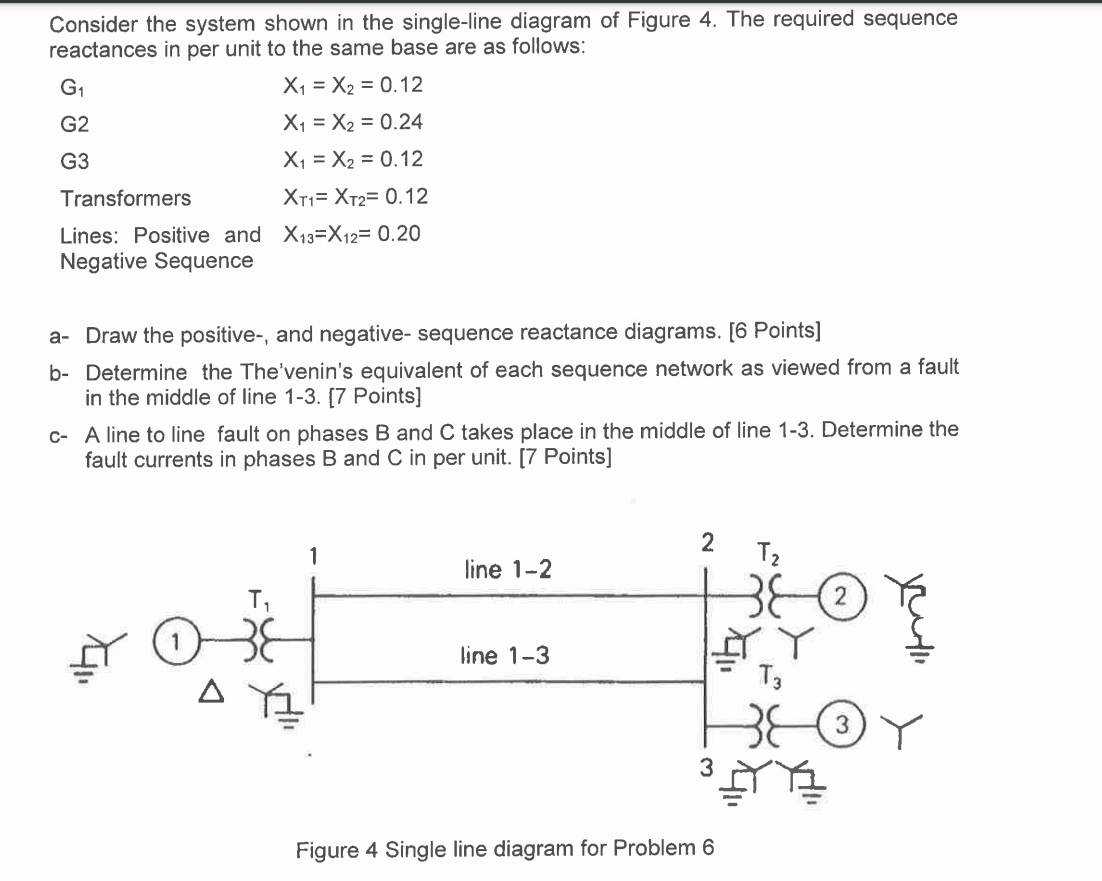Consider the system shown in the single-line diagram of Figure 4. The required sequence reactances in per unit to the same base are as follows:

G1 X1 = X2 = 0.12

G2 X1 = X2 = 0.24

G3 X1 = X2 = 0.12

Transformers X11=X12= 0.12

Lines: Positive and X13=X12= 0.20 Negative Sequence

a. Draw the positive-, and negative- sequence reactance diagrams. [6 Points]

b- Determine the The'venin's equivalent of each sequence network as viewed from a fault in the middle of line 1-3. [7 Points)

c-A line to line fault on phases B and C takes place in the middle of line 1-3. Determine the fault currents in phases B and C in per unit. [7 Points)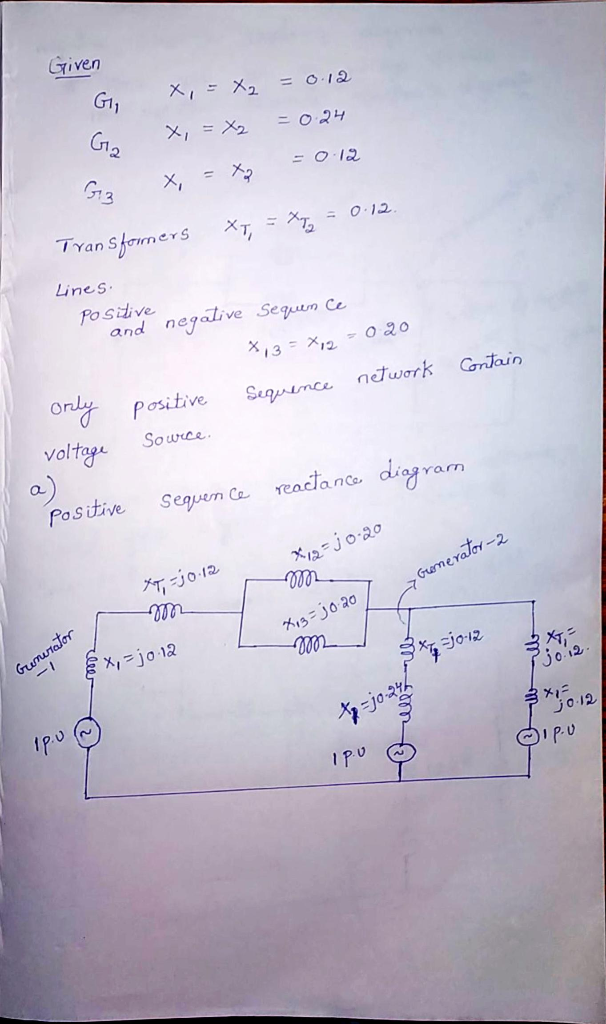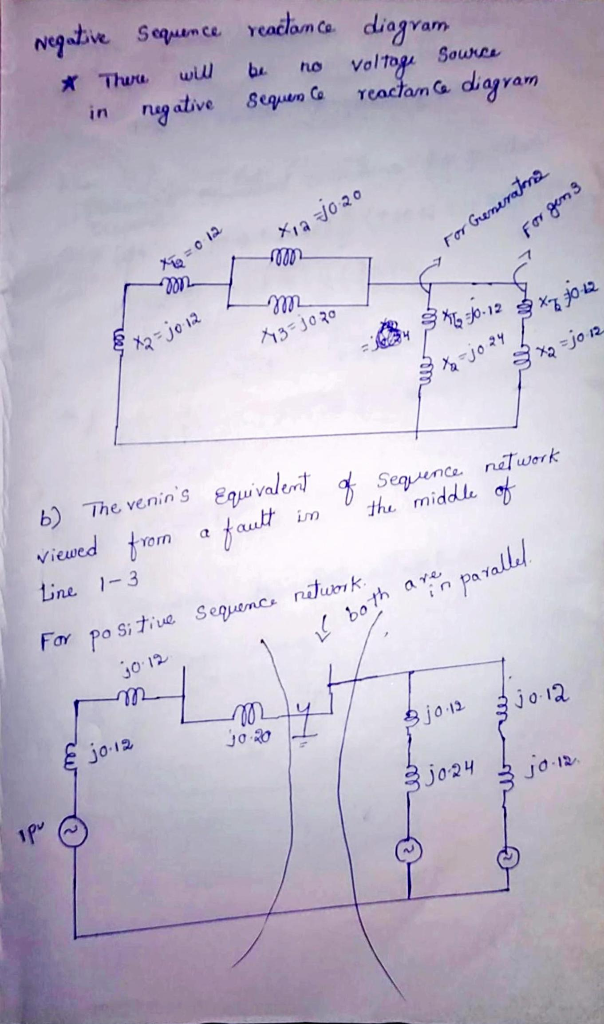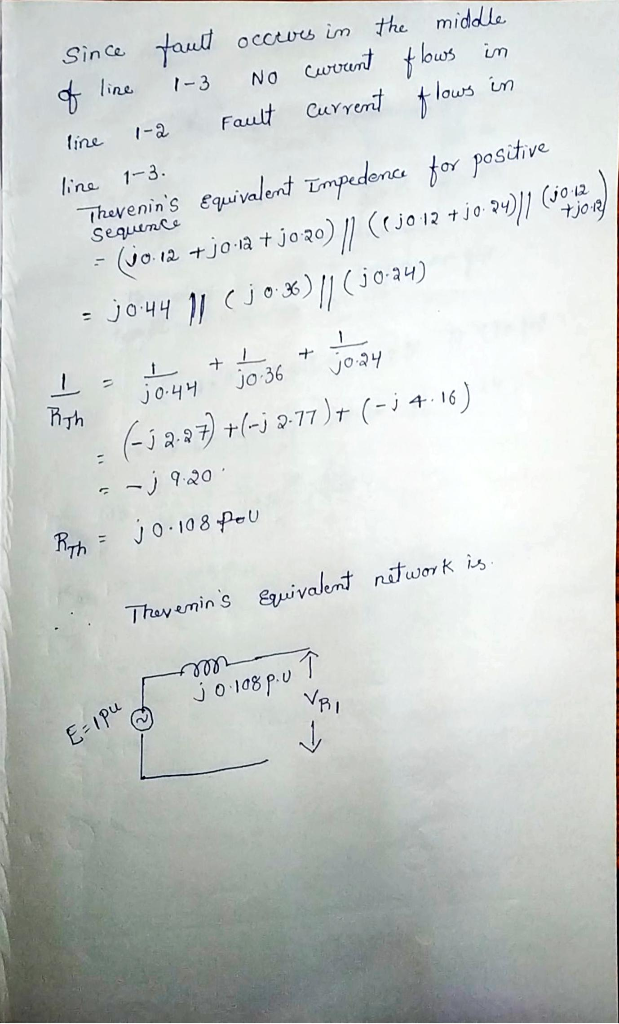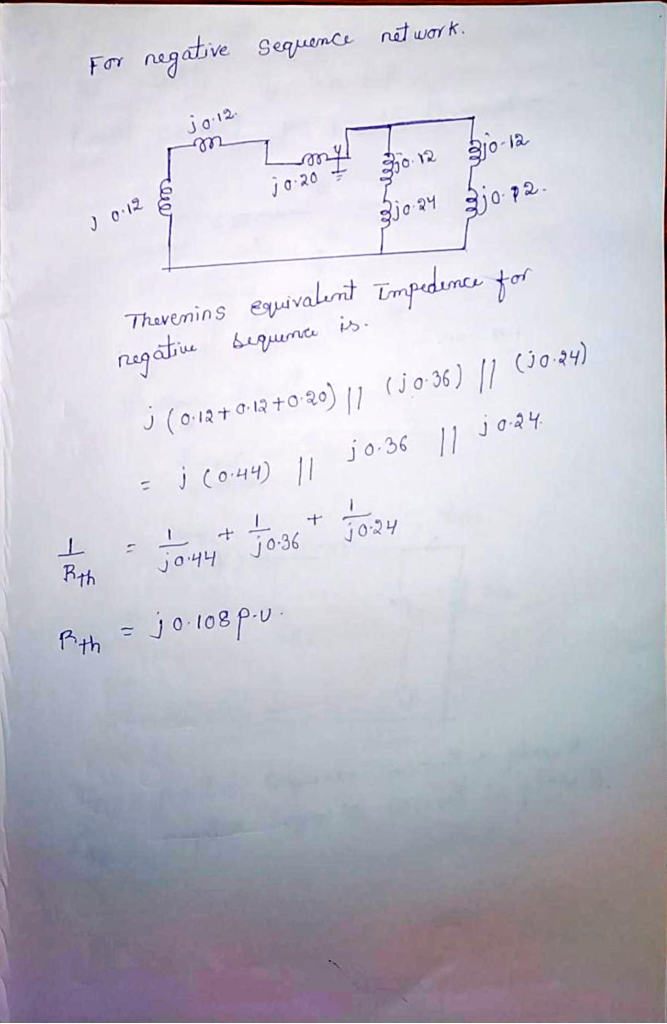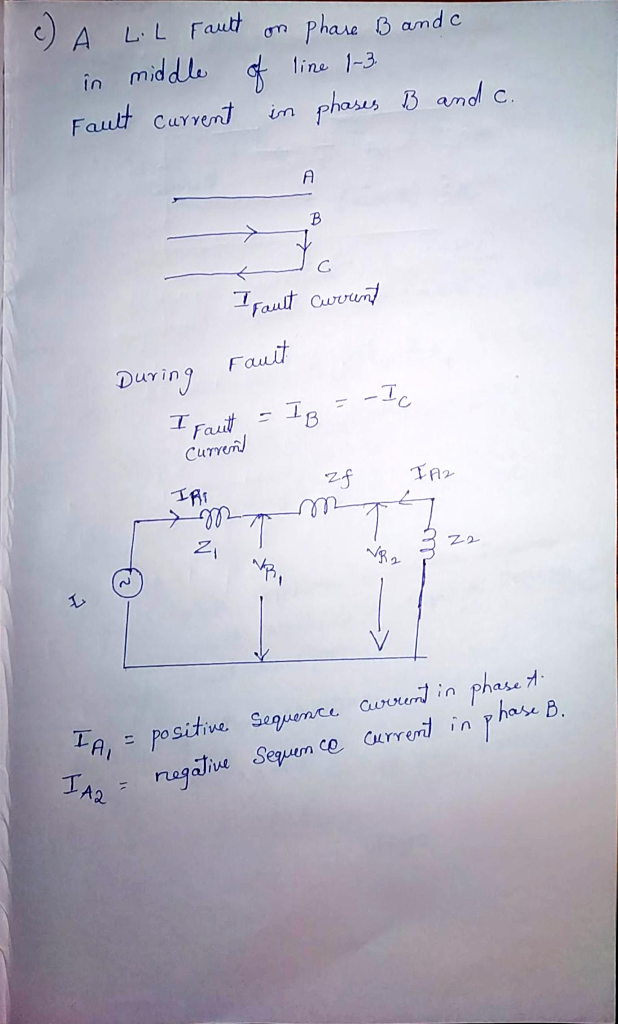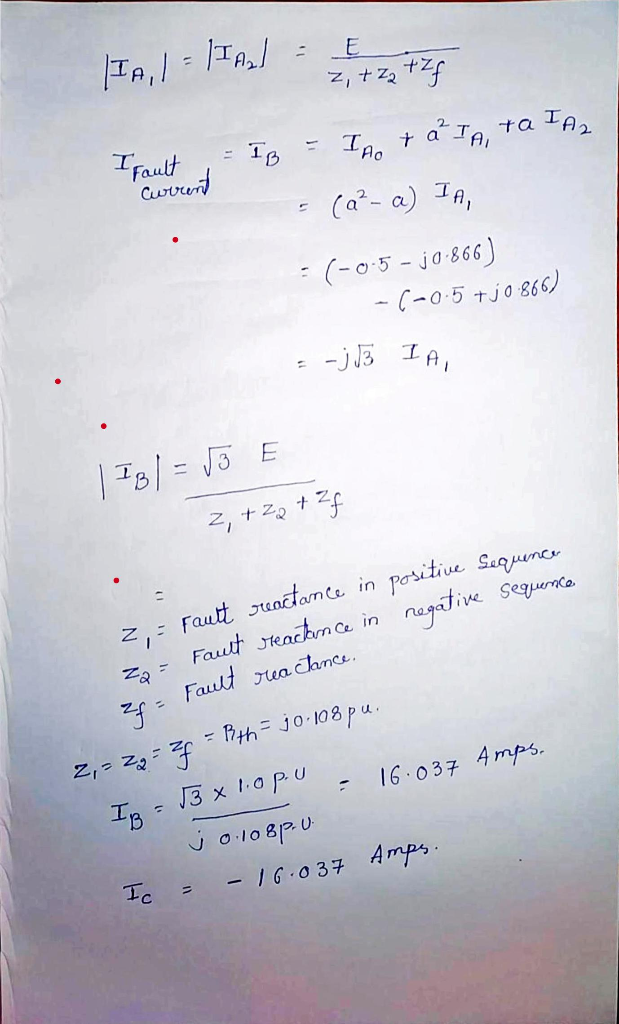IB =16.037/3

IB=-IC=5.345 Amps

#### Earn Coins

Coins can be redeemed for fabulous gifts.

Similar Homework Help Questions
• ### Q3: (15 Points) Equi below are given as follows: pment ratings and per-unit reactances for the sy...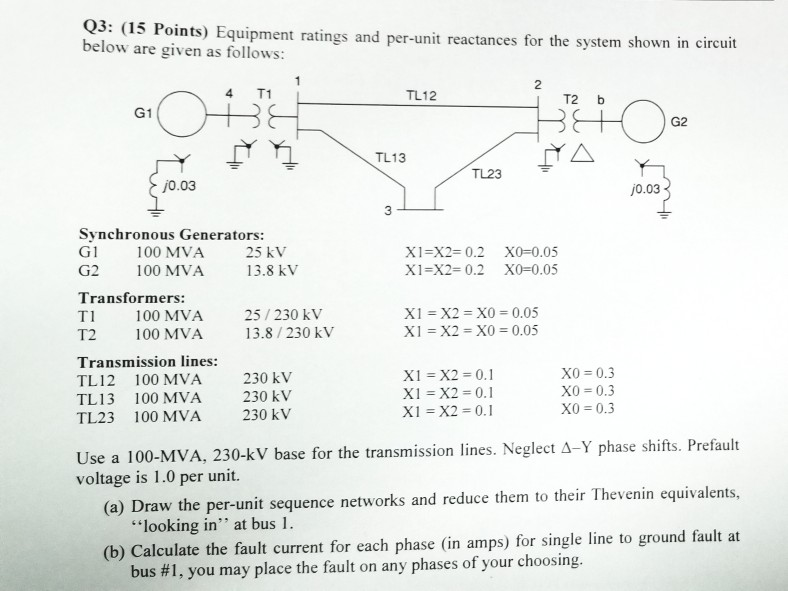Q3: (15 Points) Equi below are given as follows: pment ratings and per-unit reactances for the system shown in circuit 2 4 T1 TL12 T2b G1 G2 TL13 TL23 0.03 j0.03 Synchronous Generators: G1 100 MVA 25 kV G2 100 MVA 3.8 kV Transformers: T1 100 MVA 25/230 kV T2 100 MVA 13.8 /230 kV X1=X2= 0.2 X0-0.05 X1-X2-0.2 X0 0.05 X1 = X2-X0 = 0.05 Transmission lines: TL12 100 MVA TL13 100 MVA TL23 100 MVA X0 0.3 XO...

• ### A single line diagram of a power system is shown in Fig. 2. The system data with equipment ratings and assumed sequence reactances are given the following table. The neutrals of the generator and A-Y...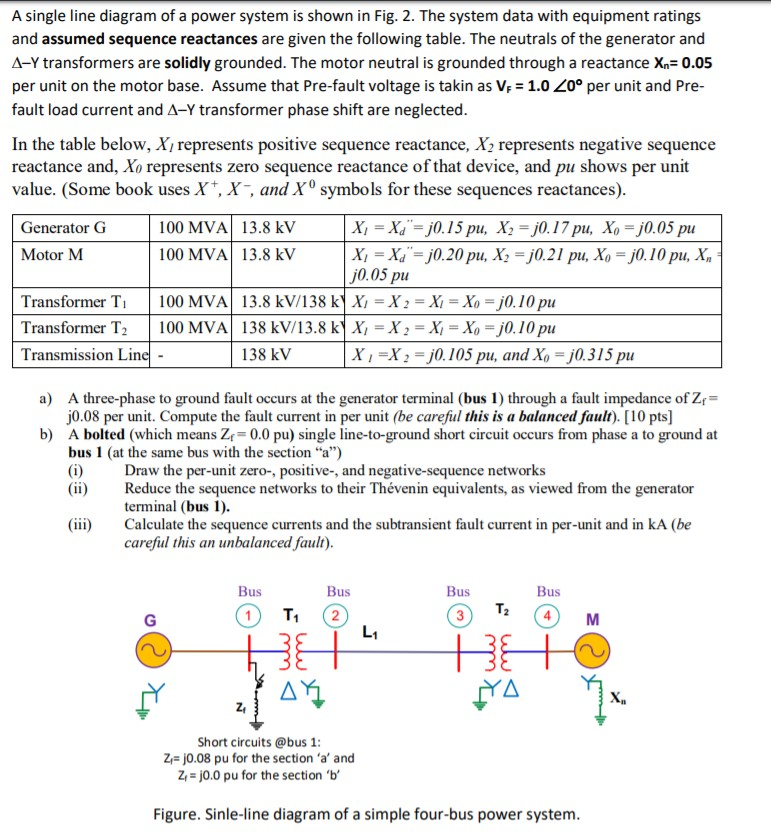A single line diagram of a power system is shown in Fig. 2. The system data with equipment ratings and assumed sequence reactances are given the following table. The neutrals of the generator and A-Y transformers are solidly grounded. The motor neutral is grounded through a reactance Xn 0.05 per unit on the motor base. Assume that Pre-fault voltage is takin as VF-1.0 ,0° per unit and Pre- fault load current and Δ-Y transformer phase shift are neglected In the...

• ### QUESTION 4. A single-line diagram of a power system is shown in Figure Q3 below, where...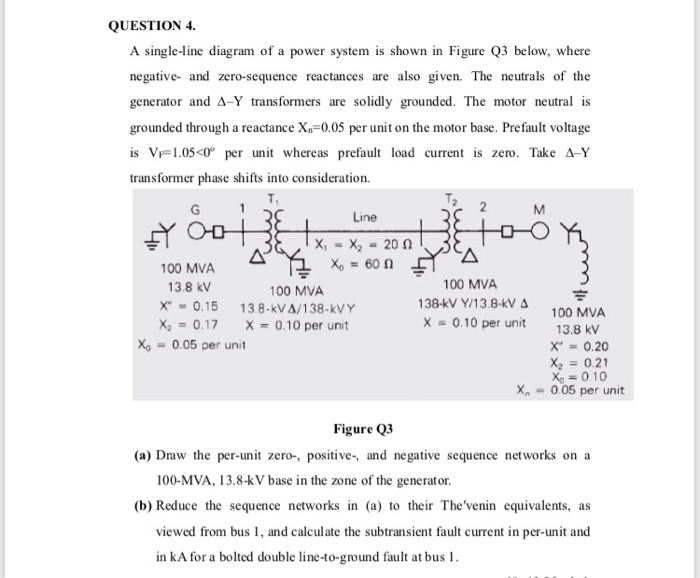QUESTION 4. A single-line diagram of a power system is shown in Figure Q3 below, where negative and zero-sequence reactances are also given. The neutrals of the generator and A-Y transformers are solidly grounded. The motor neutral is grounded through a reactance X.=0.05 per unit on the motor base. Prefault voltage is VF1.05<Oº per unit whereas prefault load current is zero. Take A-Y transformer phase shifts into consideration. M Line tool X, - X2 - 200 100 MVA X =...

• ### 03 Consider the following one line diagram. The generator is rated at 25 MVA, IN has...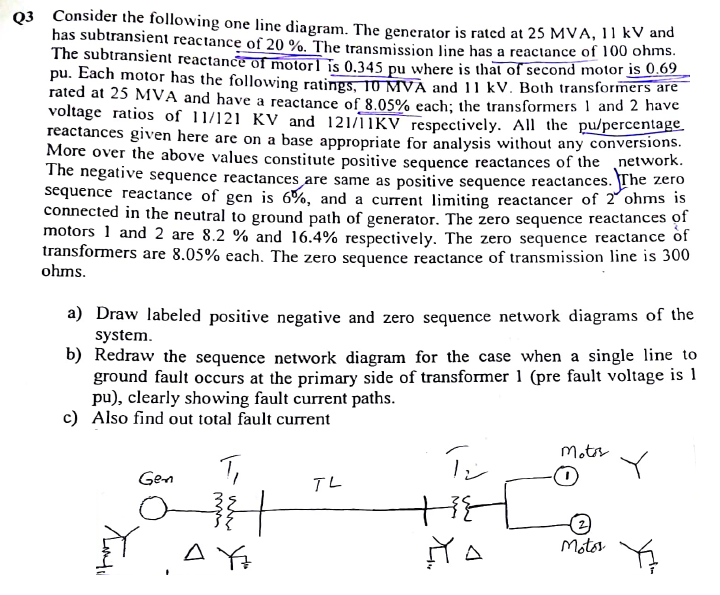03 Consider the following one line diagram. The generator is rated at 25 MVA, IN has subtransient reactance of 20 %. The transmission line has a reactance of 10 The subtransient reactance of motorlis 0.345 pu where is that ol second more pu. Each motor has the following ratings. 10 MVA and 11 kV. Both transformers are rated at 25 MVA and have a reactance of 8.05% each; the transformers 1 and 2 have voltage ratio of 11/121 KV and...

• ### 15 (c) Consider the single-line diagram of the power system shown in Figure. Neglect all resistances,...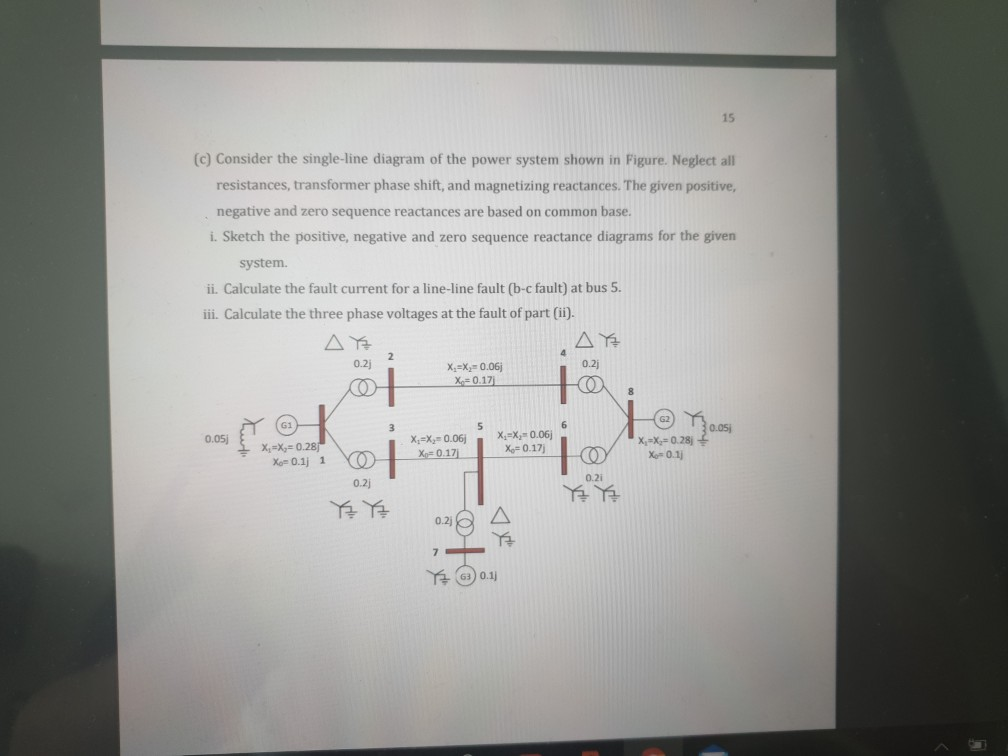15 (c) Consider the single-line diagram of the power system shown in Figure. Neglect all resistances, transformer phase shift, and magnetizing reactances. The given positive, negative and zero sequence reactances are based on common base. i. Sketch the positive, negative and zero sequence reactance diagrams for the given system. ii. Calculate the fault current for a line-line fault (b-c fault) at bus 5. iii. Calculate the three phase voltages at the fault of part (ii). AE AE 0.2) X.=X=0.06 X=0.17j...

• ### 2. A single-line diagram of the power system considered is shown in Figure P2a, where negative-...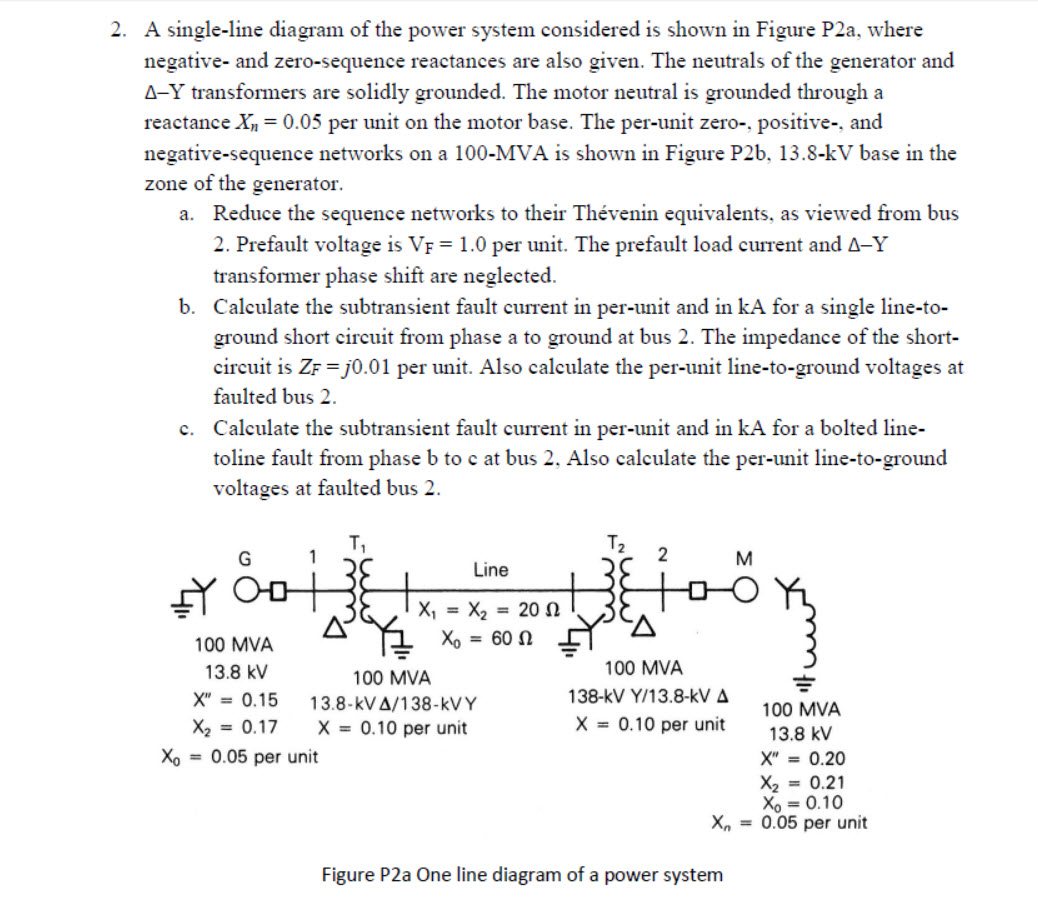2. A single-line diagram of the power system considered is shown in Figure P2a, where negative- and zero-sequence reactances are also given. The neutrals of the generator and A-Y transformers are solidly grounded. The motor neutral is grounded through a reactance Xn = 0.05 per unit on the motor base. The per-unit zero-, positive and negative-sequence networks on a 100-MVA is shown in Figure P26, 13.8-kV base in the zone of the generator. a. Reduce the sequence networks to their...

• ### Consider the system shown in the single-line diagram of Figure 3. All reactances are shown in...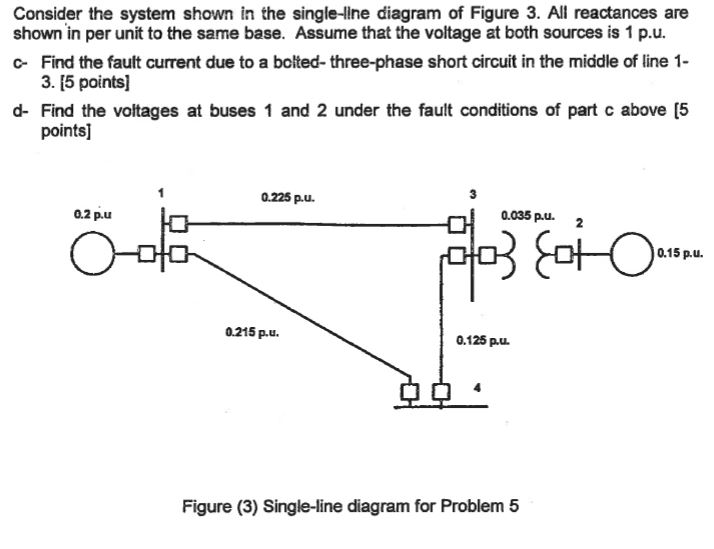Consider the system shown in the single-line diagram of Figure 3. All reactances are shown in per unit to the same base. Assume that the voltage at both sources is 1 p.u. c- Find the fault current due to a bolted-three-phase short circuit in the middle of line 1- 3. [5 points d- Find the voltages at buses 1 and 2 under the fault conditions of part c above [5 points] 0.225 pu. 0.035 pu. 0.2p.u ? 0.15 p.u. 0.215...

• ### Power System

A simple three-phase power system is shown in Figure 2. Assume that the ratings of the various devices in this system are as follows: • Generators G1, G2: 40 MVA, 13.2 kV, = 0.15 pu, = 0.15 pu, = 0.08 • Generator G3: 60 MVA, 13.8 kV, = 0.20 pu, 0.20 pu, - 0.08 • Transformers T1, T2, T3, T4: 40 MVA, 13.8/138 kV, X1 = X2 = 0.10 pu, XO 0.08 pu Transformers T5, T6: 30 MVA, 13.8/138 kV, X1 = X2...

• ### 3) The single-line diagram of a three-phase power system is shown in Fig. 1. Equipment ratings...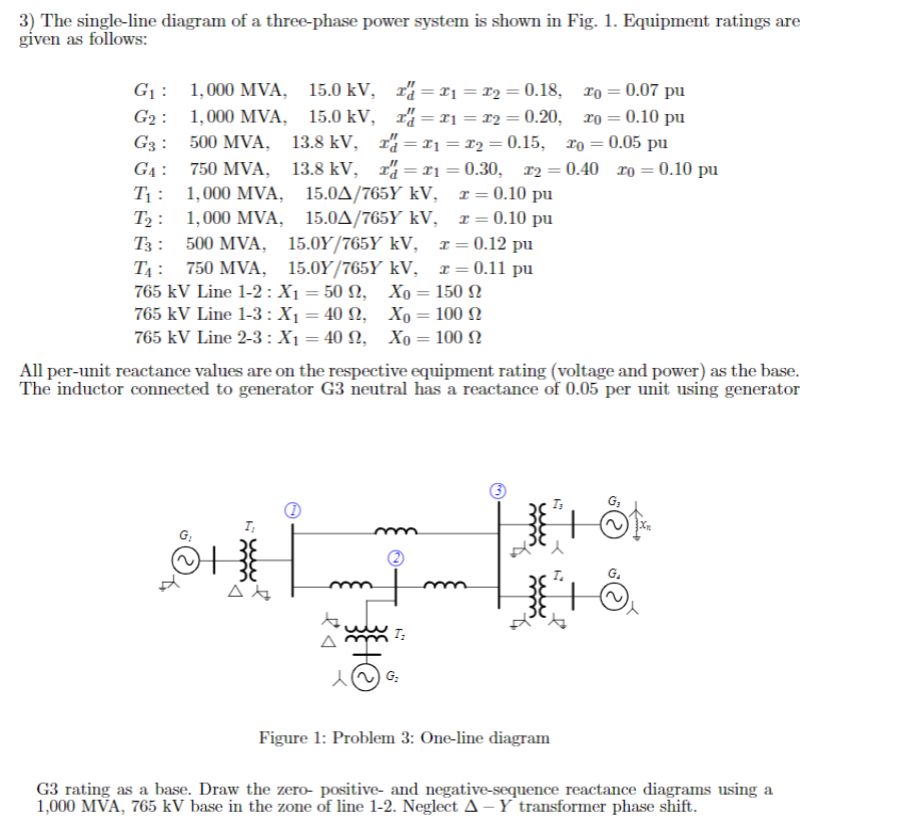3) The single-line diagram of a three-phase power system is shown in Fig. 1. Equipment ratings are given as follows: G1 1,000 MVA, 15.0 kV, 20.18, o 0.07 pu G2 : 1,000 MVA. 15.0 kV, 攻=エ1 =エ2 = 0.20, ro = 0.10 pu G3 : 500 MVA, 13.8 kV. 1" = 띠 z2 = 0.15, zo 0.05 pu G4 : 750 MVA, 13.8 kV. ェd =ェ1 = 0.30, T2 = 0.40 ro = 0.10 pu Ti : 1,000 MVA. 15.0Δ/765Y...

• ### The single-line diagram of a three-phase power system is shown. Equipment ratings are given as follows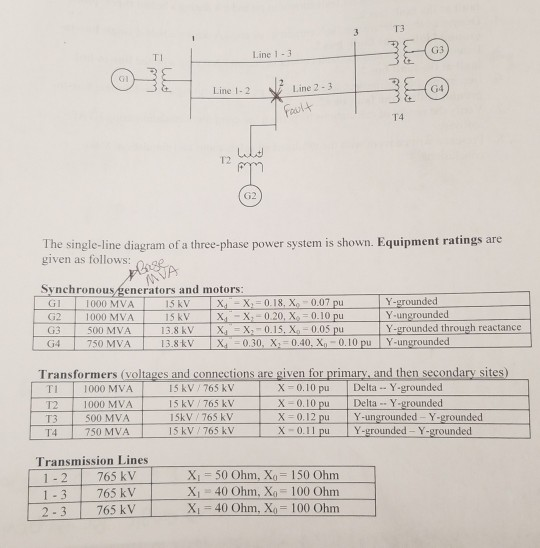The single-line diagram of a three-phase power system is shown. Equipment ratings are given as follows: The inductor connected to generator 3 neutral has a reactance of $$0.05$$ pu using generator 3 ratings as a base.1. Draw the zero-, positive-, and negative -sequence  reactance diagrams using a $$1000 \mathrm{MVA}, 765 \mathrm{kV}$$ base in the zone of line $$1-2$$.2. Faults at bus 2 are of interest. Determine the Thevenin equivalent of each sequence network as viewed from the fault bus. Prefault voltage...